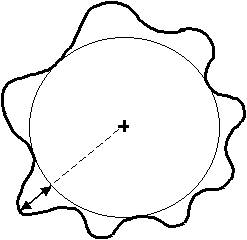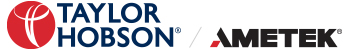Skip to content

# What reference circles are there?

Least Square Reference Circle (LSC)
The Least Squares reference circle is a circle where the sum of areas inside this circle are equal to the sum of the areas outside the circle and kept to a minimum separation.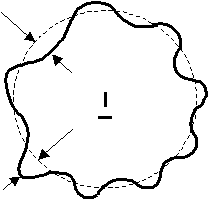The out of roundness value is the difference between the maximum and minimum radial departure from the reference circle centre.

This is a very convenient reference circle to derive, as it is mathematically precise.

Minimum Zone Circle (MZC)
The MZC is defined as two concentric circles positioned to just enclose the measured profile such that their radial departure is a minimum. The roundness value is then given as their radial separation. (RONt)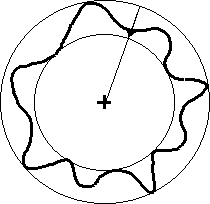Minimum Circumscribed Circle (MCC)
This is also known as the ring gauge reference circle and is the smallest circle that totally encloses the profile.
Out of roundness is quantified as the largest deviation from this circle (RONt).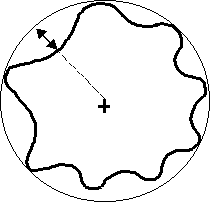Maximum Inscribed Circle (MIC)
The maximum inscribed circle, sometimes referred to as the plug gauge circle, is the largest circle that is totally enclosed by the profile. Errors are quantified as the maximum radial deviation (RONt) away from this reference circle.